# Searching Node in Binary Search Tree

### Binary Search Tree

It is a Binary Tree in which the value of the left child of a Node is always less than the value of the Node and value of right child is always greater than the value of the Node.

Look at the example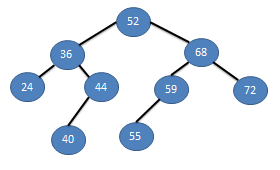NOTE : Inorder Traversal of BST gives you a sorted list of elements

I hope you know what a Binary Search Tree (BST) is. Now performing Searching Operation in BST is finding a specific value from the tree.

Steps involve are :

1. Make currentNode –> rootNode
2. If currentNode = null
3. Compare value
• If value==currentNode’s value ==> Found, exit
• If value < currentNode's value ==> currentNode = its left child, Go to step 2
• If value > currentNode’s value ==> currentNode = its right child, Go to step 2

### Still have Doubt ??? Let’s take an example and Dry Run it step by Step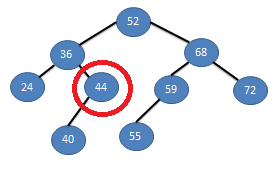From the above tree lets Suppose you are searching for value 44.
Now start from the Root and compare values.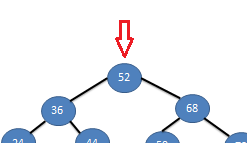As 44 is smaller than 52, so go to is left child. and compare.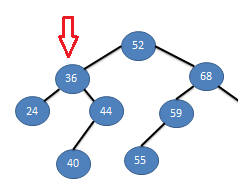Now as 44 is greater than 36, we go to its right child.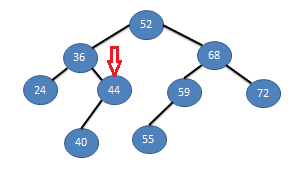Compare values , Yo we found it. !!!!